### A basic terrain generator in Go (Part 4)

Cliffs of Dover

In the last part, we added fancy output code, and our output looks like this now: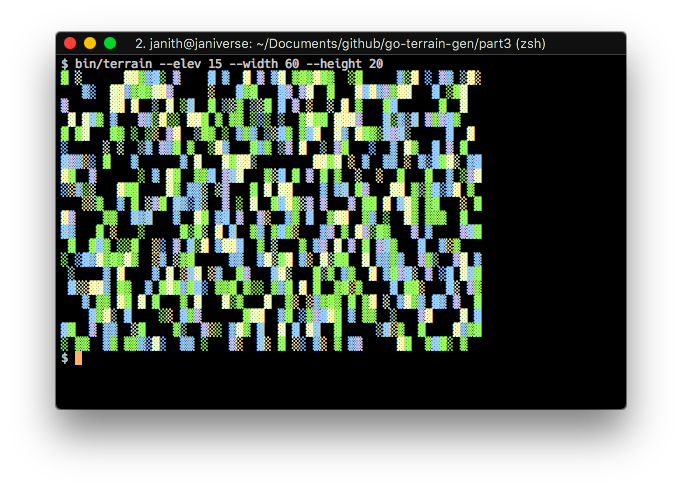...which, if you've ever seen a map, isn't what terrain actually looks like. The terrain around peaks tend to be at a similar height, and there is rarely the random patchwork of heights we see here. So let's fix that!

But first...

### Visualise Steps

Wouldn't it be cool to see how the terrain gets generated layer by layer from the highest elevation to the lowest? Let's change our `Generate()` method to allow us to view this.

```func (f *fullMap) Generate(viewSteps bool) {
// rand needs to be seeded, so we set the current
// nanosecond timestamp as the seed
rand.Seed(time.Now().UnixNano())

// iterate down from max elevation, assigning vals
for e := f.elevation; e > 0; e-- {
for h := 0; h < f.height; h++ {
for w := 0; w < f.width; w++ {
// if the element has already been assigned, skip it
if f.elements[h][w] > 0 {
continue
}

// if the random value meets our criteria, it's a peak
if rand.Intn(100) < f.peakProbability {
f.elements[h][w] = e
}
}
}

// if viewSteps is true, we print every step of the generation process,
// sleep for a second, and clear the screen for the next step
if viewSteps {
f.Print()
time.Sleep(time.Second)
exec.Command("clear")
fmt.Println()
}
}
}
```

Let's also add it as a CLI flag in our `main.go` so that the user can turn it on when they want.

```func main() {
height := flag.Int("height", 16, "height of the map")
width := flag.Int("width", 16, "width of the map")
elevation := flag.Int("elev", 9, "levels of elevation on the map")
peakProbability := flag.Int("peaks", 5, "percentage probability a peak will randomly appear")
steps := flag.Bool("steps", false, "show the generation steps")
flag.Parse()

terrainMap := terrain.NewFullMap(*width, *height, *elevation, *peakProbability)
terrainMap.Generate(*steps)
terrainMap.Print()
}
```

Now, we can compile and run with the `--steps` flag to view our little generator in action: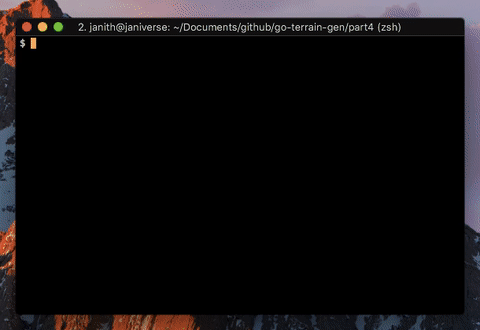Groovy! 💃🏽

### Neighbours

Like we discussed before, the map elements next to peaks need to be at a similar height. My logic for this part is going to be: if the element next to you is at elevation e, then your elevation is e - 1. To take a really simple example, elevation values would look like this around a peak: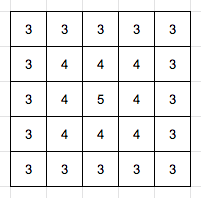We'll refine this later (adding cliffs!), but for now, it looks like we need to find if you have an element adjacent to you at an elevation `e`, so we'll define a new unexported method:

```// adjacentElevation checks if an adjacent element
// to the given element (h, w) is at a given elevation
func (f *fullMap) adjacentElevation(h, w, elevation int) bool {
for y := max(0, h-1); y <= min(f.height-1, h+1); y++ {
for x := max(0, w-1); x <= min(f.width-1, w+1); x++ {
if f.elements[y][x] == elevation+1 {
return true
}
}
}

return false
}
```

Go's `Min()` and `Max()` methods, as provided by the `math` package, work with `float64` numbers, so we'll also define two simple private functions to work with `int`s and get min and max values:

```func min(x, y int) int {
if x < y {
return x
}
return y
}

func max(x, y int) int {
if x > y {
return x
}
return y
}
```

Now, let's modify the `Generate()` method to use `adjacentElevation()`:

```// Generate generates the terrain map
func (f *fullMap) Generate(viewSteps bool) {
// rand needs to be seeded, so we set the current
// nanosecond timestamp as the seed
rand.Seed(time.Now().UnixNano())

// iterate down from max elevation, assigning vals
for e := f.elevation; e > 0; e-- {
for h := 0; h < f.height; h++ {
for w := 0; w < f.width; w++ {
// if the element has already been assigned, skip it
if f.elements[h][w] > 0 {
continue
}

// if the element is next to a element with elevation x, it
// should get elevation x - 1
// alternately, if the random value meets our criteria, it's a peak
if f.adjacentElevation(h, w, e) || rand.Intn(100) < f.peakProbability {
f.elements[h][w] = e
}
}
}

// if viewSteps is true, we print every step of the generation process,
// sleep for a second, and clear the screen for the next step
if viewSteps {
f.Print()
time.Sleep(time.Second)
exec.Command("clear")
fmt.Println()
}
}
}
```

Let's compile and run it!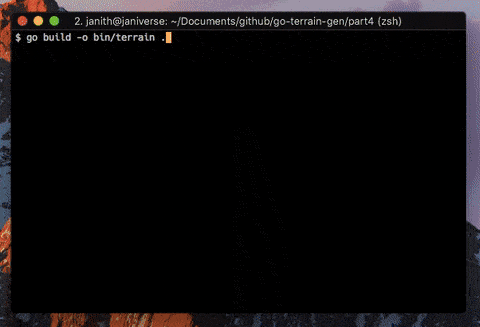I think it's starting to look a lot like a real map now. 🗺

### Cliffs

We know that in the real world, mountains aren't all shaped like this:Sometimes, they look like this too:Also, things are looking a wee bit too boxy, so let's add some randomness in the form of a cliff probability1 to the `fullMap` struct and the `NewFullMap()` function:

```type fullMap struct {
width, height, elevation, peakProbability, cliffProbability int
elements                                                    [][]int
}

// NewFullMap returns a new terrain map
func NewFullMap(width, height, elevation, peakProbability, cliffProbability int) fullMap {
elements := make([][]int, height)
for i := 0; i < height; i++ {
elements[i] = make([]int, width)
}

return fullMap{
width:            width,
height:           height,
elevation:        elevation,
peakProbability:  peakProbability,
cliffProbability: cliffProbability,
elements:         elements,
}
}
```

We'll also edit `main.go` to take `--cliffs` as a CLI flag:

```func main() {
height := flag.Int("height", 16, "height of the map")
width := flag.Int("width", 16, "width of the map")
elevation := flag.Int("elev", 9, "levels of elevation on the map")
peakProbability := flag.Int("peaks", 5, "percentage probability a peak will randomly appear")
cliffProbability := flag.Int("cliffs", 5, "percentage probability a cliff will randomly appear")
steps := flag.Bool("steps", false, "show the generation steps")
flag.Parse()

terrainMap := terrain.NewFullMap(*width, *height, *elevation, *peakProbability, *cliffProbability)
terrainMap.Generate(*steps)
terrainMap.Print()
}
```

Let's now edit `adjacentElevation()` to use cliffProbability:

```func (f *fullMap) adjacentElevation(h, w, elevation int) bool {
for y := max(0, h-1); y <= min(f.height-1, h+1); y++ {
for x := max(0, w-1); x <= min(f.width-1, w+1); x++ {
if f.elements[y][x] == elevation+1 {
// if this element is *not* randomly a cliff, return true
return rand.Intn(100) > f.cliffProbability
}
}
}

return false
}
```

Okay, now to compile and run: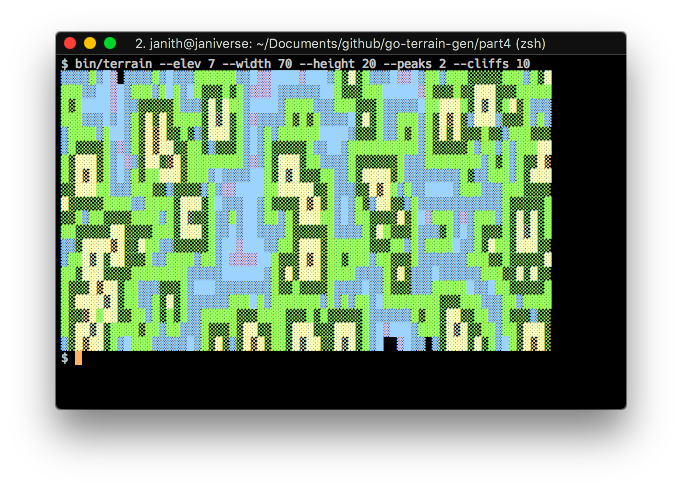Woo! 🥂 That looks much more realistic, and by tweaking around the peak and cliff probabilities, and the number of elevation levels, we can get maps that looks really nice.

So that concludes this long (and I hope educational) series on using Go to write a simple terrain generator on the CLI. I hope you enjoyed following along as much as I enjoyed coding and writing about it!

The full code, as always, can be found on Github.

1. Just like our old friend peak probability, this is the random percentage of the probability of an element being a cliff.

Built using Pelican.